MATHEMATICS

 Time: Two Hours  (8.30 AM – 10.30 AM) Max.Marks: 60

NOTE:-

1. Attempt all questions.
2. Rough work must be enclosed with answer book.
3. While answering, refer to a question by its serial number as well as section heading. (eg.Q2/Sec.A)
4. There is no negative marking.
5. Answer each of Sections A, B, C at one place.
6. Elegant solutions will be rewarded.
7. Use of calculators, slide rule, graph paper and logarithmic, trigonometric and statistical tables is not permitted.

Note:- All answers to questions in Section-A, Section-B and Section-C must be supported by mathematical arguments. In each of these sections order of the questions must be maintained.

SECTION-A

This section has Six Questions. Each question is provided with five alternative answers. Only one of them is the correct answer. Indicate the correct answer by A, B, C, D, E.

(6x2=12 MARKS)

1. Real numbers x1, x2, …., x2007 are chosen such that (x1, x2), (x2, x3), …., (x2006, x2007) are all points on the graph of y =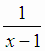A) Such a choice is possible for all real x1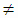1
B) In every choice x1, x2, …, x2007 are all distinct
C) There are infinitely many choices in which all xi’s are equal
D) There exists a choice in which the product x2.x3…..x2007 = 0
E) None of these

2. The consecutive sides of an equiangular hexagon measure x, y, 2, 2006, 3, 2007 units

A) The hypothesis never takes place
B) The greatest side measures 2007 units and the smallest 2
C) The greatest side measures 2007 units, but the smallest x
D) The greatest side measures y units, but the smallest 2
E) The smallest side measures x units, the greatest y

3. ABCD is a convex quadrilateral

A) A circle can always be circumscribed to it
B) A circle can never be circumscribed to it
C) A circle can always be inscribed in it
D) A circle can never be inscribed in it
E) None of these

4. A lattice point in a plane is one both of whose coordinates are integers. Let O be (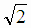, 1) and P any given lattice point. Then the number of lattice points Q, distinct from P, such that OP = OQ is

A) 0     B) 1    C) not necessarily 0, not necessarily 1, but either 0 or 1    D) infinitely many     E) none of these

5. i) f(x, y) is the polynomial f0(x)y2007 + f1(x)y2006 + f2(x)y2005 + ….+ f2006(x)y + f2007(x), where each fi(x) is a polynomial in x with real coefficients, and ii) (x-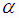) is a factor of f(x, y), whereis a real number. Then

A) there exists a f(x, y) such that fi(x) = x2 + 1 for some i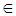{ 0, 1, 2, …, 2007}
B) there exists a f(x, y) such that fi(x) = x -+ 1 for some i{ 0, 1, 2, …, 2007}
C) there exists a f(x, y) such that fi(x) = (x-)2006 + 1 for some i{ 0, 1, 2, …, 2007}
D) there exists a f(x, y) such that f0(x) = x2 –(2+1)x + (2+)
E) none of these

6. (b-c)(x-a)(y-a) + (c-a)(x-b)(y-b) + (a-b)(x-c)(y-c) is

A) independent of x, but not of y     B) independent of y, but not of x
C) independent of both x and y     D) independent neither of x, nor of y
E) independent of x only if not independent of y

SECTION-B

This section has Six Questions. In each question a blank is left. Fill in the blank.

(6x2=12 MARKS)

1. For the purpose of this question, a square is considered a kind of rectangle. Given the rectangle with vertices (0, 0), (0, 223), (9, 223), (9, 0), divided into 2007 unit squares by horizontal and vertical lines. By cutting off a rectangle from the given rectangle, we mean making cuts along horizontal and (or) vertical lines to produce a smaller rectangle. Let m be the smallest positive integer such that a rectangle of area ‘m’ cannot be cut off from the given rectangle. Then m = _________

2. A line has an acute angled inclination and does not pass through the origin. If it makes intercepts a and b on x-, y-axes respectively, then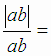_________

3. If k is a positive integer, let Dk denote the ultimate sum of digits of k. That is, if k is a digit, then Dk = k. If not, take the sum of digits of k. If this sum is not a single digit, take the sum of its digits. Continue this process until you obtain a single digit number. By Dk we mean this single digit number. {Dp / p is a positive multiple of 2007} = _________, in roster form.

4. The digits of a positive integer m can be rearranged to form the positive integer n such that m+n is the 2007-digited number, each digit of which is 9. The number of such positive integers m is ________.

5.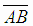and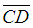are chords of a circle such that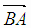and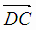intersect in a point E outside the circle. F is a point on the minor arc BD such that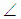FAB = 220,FCD = 180. ThenAEC +AFC = _________.

6. The quadratic ax2 + bx + a = 0 has a positive coincident root. Then= _________.

SECTION-C

(6x2=12 MARKS)

1. Explain a way of subdividing a 102 X 102 square into 2007 non-overlapping squares of integral sides.

2. ABC is a triangle. Explain how you inscribe a rhombus BDEF in the triangle such that D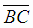, E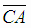and F.

3. Equilateral triangle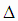ABC has centroid G. A1, B1, C1 are points on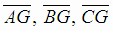such that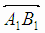,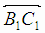,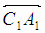are respectively parallel to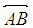,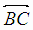,  If the distance betweenandis one-sixth of the altitude ofABC, determine the ratio of areas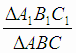.

4. P(x) is a polynomial in x with real coefficients. Given that the polynomial P2(x) + (9x-2007)2 has a real root, determineand also the multiplicity of.

5. Find the homogeneous function of 2nd degree in x, y, which shall vanish when x = y and also when x=4, y=3 and have value 2 when x = 2, y = 1.

6. If 3yz + 2y + z + 1 = 0 and 3zx + 2z + x + 1 = 0, then prove that 3xy + 2x + y + 1 = 0.

SECTION-D

(6x4=24 MARKS)

1. x3 is the 753rd AM of 2007 AM’s inserted between x1 and x2. y3 is the 753rd AM of 2007 AM’s inserted between y1 and y2. Show that A(x1, y1), P(x3, y3), B(x2, y2) are collinear. Determine also the ratio AP : PB.

2. Lines l and m intersect in O. Explain how you will construct a triangle OPQ such that Pl, Qm,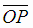and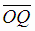are equal in length and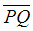is of given length ‘a’.

3. i)ABC = 1200. ii)ACD is equilateral, iii) B and D are on opposite sides of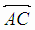. Prove that a)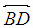bisectsABC and b)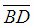is in length equal to the sum of lengths ofand.

4. a1, a2, …, a2007 are 1, 2, …, 2007 in some order. If x is the greatest of 1.a1, 2.a2, …, 2007.a2007, prove that x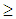(1004)2.

5. Prove that for all integers n2, 2n-1 ( 3n + 4n ) > 7n.

6. Resolve x8 + y8 into real quadratic factors.